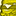# App Development

Announcements
Now Live: Qlik Sense SaaS Simplified Authoring – Analytics Creation for Everyone: READ DETAILS
cancel
Showing results for
Did you mean:Contributor III

## Count if with count if condition

Hello everyone,

I wonder if it possible to do a count if with a count if condition.

Here is a table showing people doing differents activities :

 Name Activity Jean Football Jean Cinema Jean Jogging Jean Beer Marie Tennis Marie Cinema Marie Boxe Daniel Jogging Cecile Golf Cecile Tennis Cecile Cinema Cecile Shopping Cecile Jogging Nathan Tennis Nathan Judo Nathan Cinema

I want to know the number of people who have exactly 3 activities. The results is supposed to be 2 since there are 2 people who have exactly 3 activities (Marie and Nathan).

I believe a count if with a set analysis is the way to do it, but I can't manage to make it work.

Here what I tried : Count(distinct{<Count(distinct[Activity])='3'>}[Name])

Does anyone know if there is formula to solve this ? Thank in advance.

Best regards.

Labels (2)

• ### Count if condition

1 Solution

Accepted SolutionsMVP

Try this

Count(DISTINCT {< Name = {"=Count(DISTINCT [Activity]) = 3"}>} [Name])

7 RepliesMVP

Try this

Count(DISTINCT {< Name = {"=Count(DISTINCT [Activity]) = 3"}>} [Name])Contributor III
Author

Thank you, it works.Contributor II

This could help with something I'm currently stuck on. Could the =3 be replaced by >variable? I'm having no luck with thatMVP

I would think so... can you share an example of where this doesn't work?Contributor II

Hi Sunny, so using your original solution I am able to make this work:

=Count(DISTINCT {< [Customer ID] = {"=

(sum({\$<[Sales Type] = {'Classified'},UniqueEvent={'view'}>}EventCount)/sum({\$<[Sales Type] {'Classified'}>}VacancyCount))

>

180

"}>} [Customer ID])

and that counts the number of customers where the calculation is greater than 180

What I can't manage is replacing the 180 with a second calculation so it looks like this:

=Count(DISTINCT {< [Customer ID] = {"=

(sum({\$<[Sales Type] = {'Classified'},UniqueEvent={'view'}>}EventCount)/sum({\$<[Sales Type] {'Classified'}>}VacancyCount))

>

sum({\$<[Customer ID]= E([Customer ID]),[Sales Type] = {'Classified'},UniqueEvent={'view'}>}EventCount)/sum({\$<[Customer ID]= E([Customer ID]),[Sales Type] = {'Classified'}>}VacancyCount)

"}>} [Customer ID])

that gives me 0, even though the value of the second calculation in this case = 180MVP

May be you need TOTAL qualifier

``````=Count(DISTINCT {< [Customer ID] = {"=

(Sum({\$<[Sales Type] = {'Classified'}, UniqueEvent = {'view'}>} EventCount)/Sum({\$<[Sales Type] = {'Classified'}>} VacancyCount))

>

Sum(TOTAL {\$<[Customer ID] = E([Customer ID]), [Sales Type] = {'Classified'}, UniqueEvent = {'view'}>} EventCount)/Sum({\$<[Customer ID] = E([Customer ID]),[Sales Type] = {'Classified'}>} VacancyCount)

"}>} [Customer ID])``````Contributor II

That worked, thanks Sunny 🙂

For anyone else wondering, in my case I changed both the numerator and the denominator from sum( to sum(total, though perhaps doing just one would workTags